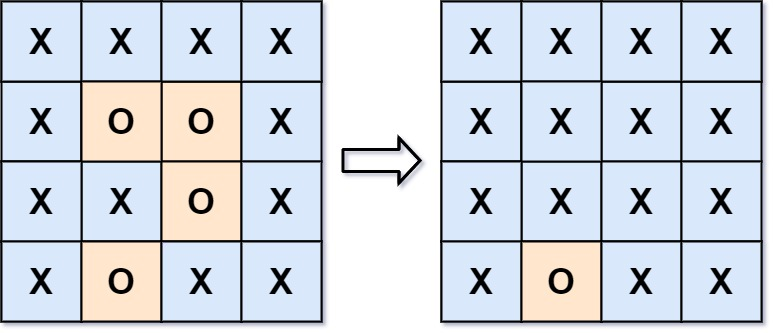130. Surrounded Regions
Medium
7.1K
1.5K

Given an `m x n` matrix `board` containing `'X'` and `'O'`, capture all regions that are 4-directionally surrounded by `'X'`.

A region is captured by flipping all `'O'`s into `'X'`s in that surrounded region.

Example 1:```Input: board = [["X","X","X","X"],["X","O","O","X"],["X","X","O","X"],["X","O","X","X"]]
Output: [["X","X","X","X"],["X","X","X","X"],["X","X","X","X"],["X","O","X","X"]]
Explanation: Notice that an 'O' should not be flipped if:
- It is on the border, or
- It is adjacent to an 'O' that should not be flipped.
The bottom 'O' is on the border, so it is not flipped.
The other three 'O' form a surrounded region, so they are flipped.
```

Example 2:

```Input: board = [["X"]]
Output: [["X"]]
```

Constraints:

• `m == board.length`
• `n == board[i].length`
• `1 <= m, n <= 200`
• `board[i][j]` is `'X'` or `'O'`.
Accepted
537.2K
Submissions
1.5M
Acceptance Rate
37.0%

Seen this question in a real interview before?
1/4
Yes
No

Discussion (0)

Related Topics